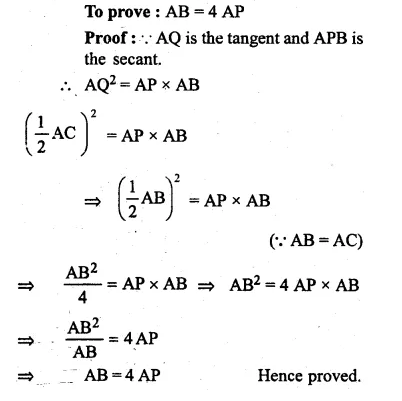Guru

# Question 33. (a) In the figure (i) given below, PAB is secant and PT is tangent to a circle. If PA : AB = 1:3 and PT = 6 cm, find the length of PB. (b) In the figure (ii) given below, ABC is an isosceles triangle in which AB = AC and Q is mid-point of AC. If APB is a secant, and AC is tangent to the circle at Q, prove that AB = 4 AP

• -1

This is the basic and conceptual question from Chapter name- circles
Topic – Angle properties of circles
Chapter number- 15

In this question we have a figure(i)

in which , PAB is secant and PT is tangent to a circle.

It is given that PA : AB = 1:3 and PT = 6 cm, now we have to find the length of PB.

(b) In the figure (ii) given in the question ABC is an isosceles triangle in which AB = AC and Q is mid-point of AC.

APB is a secant, and AC is tangent to the circle at Q,

Now we are asked to prove that AB = 4 AP

ICSE Avichal publication
Understanding ICSE Mathematics
Question 33

Share